The Inner Product, March 2002
Jonathan Blow (jon@number-none.com)
Last updated 17 January 2004

Hacking Quaternions

Related articles:

IK with Quaternion Joint Limits (April 2002)
Understanding Slerp, Then Not Using It (April 2004)

Quaternions are a nifty way to represent rotations in 3D space. You can find many introductions to quaternions out there on the internet, so I'm going to assume you know the basics. For a refresher, see the papers by Shoemake or Eberly in the References.

In this article we will look closely at the tasks of quaternion interpolation and normalization, and we'll develop some good tricks.

Interpolation

When game programmers want to interpolate between quaternions, they tend to copy Ken Shoemake's code without really understanding it. Ken uses a function called slerp that walks along the unit sphere in 4-dimensional space from one quaternion to the other. Because it's navigating a sphere, it involves a fair amount of trigonometry, and is correspondingly slow.

Lacking a strong grasp of quaternions, most game developers just accept this: slerp is slow, and if you want something faster, maybe you should go back to Euler angles and all their nastiness. But the situation is not so bad. There's a cheap approximation to slerp that will work in most cases, and is so braindead simple and fast that it's shocking. Shocking, I tell you.

What Slerp Does

Slerp is desirable because of two main properties; any approximation we formulate would ideally have the same properties. The first, and perhaps most important, is that slerp produces the shortest path between the two orientations on that unit sphere in 4D; this is equivalent to finding the "minimum torque" rotation in 3D space, which you can think of as the smoothest transition between two orientations. The second property of slerp is that it travels this path at a constant speed, which basically means you have full control over the nature of the transition (if you want to add some style, like starting slowly and then speeding up, you can just spline your time parameter before feeding it into slerp.)

So here's our approximation: linearly interpolate the two quaternions, componentwise. That is, if t is your time parameter from 0 to 1, then x = x0 + t (x1 - x0), and similarly for y, z, and w.

One might say: Ridiculous! How could that possibly be a worthwhile interpolation, when the "right answer" is so much more complicated? Let's take a look.

Figure 1 shows a 2-dimensional version of quaternion interpolation. Slerp walks around the edge of the unit circle, which is what we want. Linear interpolation results in a chord that cuts inside the circle. But here's the thing to realize: normalizing all the points along the chord stretches them out to unit length, so that they lie along the slerp path. To restate: if you linearly interpolate two quaternions from t = 0 to t = 1, and then normalize the result, you get the same minimal-torque transition that slerp would have given you.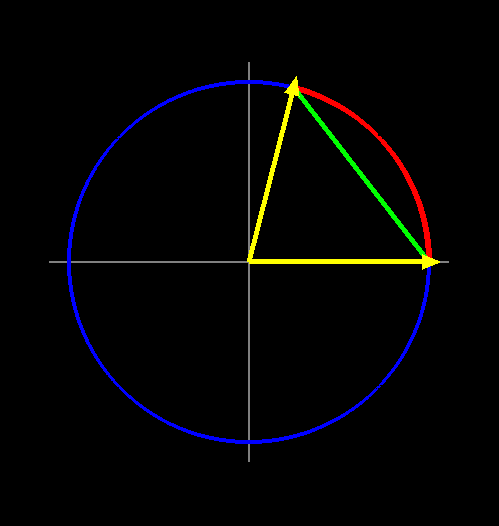Figure 1: A 2-dimensional picture of quaternion interpolation. The blue circle is the unit sphere; the two yellow vectors are the quaternions. The red arc represents the path traveled by slerp; the green chord shows the path taken by linear interpolation.

The Linear Algebra way to see this is that both the great circle and the chord lie in Span(q0, q1), which is a 2D subspace of the 4D embedding space. Adding the constraint that Length(Interpolate(q0, q1, t)) = 1 reduces the dimensionality to one, so both paths must lie along the same circle. And both forms of interpolation produce only a continuous path of points between q0 and q1, so they must be the same.

If q0 and q1 lie on opposing points of the sphere, the chord will pass through the origin and normalization will be undefined. But that's okay -- unless you're doing something wacky, you don't want your quaternions to be further than 90 degrees apart in the first place. (Because every rotation has 2 quaternion representations on the unit sphere, and you want to pick the closest ones to interpolate between.) So the normalization will always be well-defined.

So the normalized linear interpolation and the slerp both trace out the same path. There is a difference between them, though: they travel at differing speeds. The linear interpolation will move quickly at the endpoints and slowly in the middle. Figure 2 shows a graph of the worst case, 90 degrees.

The function graphed in Figure 2 iswhere α is the original angle between the two quaternions. I figured this out just by drawing a 2D graph like Figure 1, where one of my vectors is the x axis (1, 0) and the other one is (cos α, sin α). Then I just wrote an expression for linearly interpolating between them by 't', and then finding the resulting angle by tan-1. This rather simplistic approach is valid because: (a) since all the action happens in Span(q0, q1), we can just take that 2D cross-section out of 4D space; studying it in isolation, we see the entirety of what is happening. And (b) on the resulting 2D unit circle, because the set of all possibilities for the two unit vectors is redundant by rotational symmetry, we can choose one of the vectors to be anything we like; I chose (1, 0) to simplify the math.

Casey Muratori is the first person I know of who considered linear interpolation of quaternions as a serious option. He investigated numerically and found linear interpolation, when properly employed, to be quite worthwhile. So hats off to Casey, eh? Casey makes the animation toolkit Granny 2 (by RAD Game Tools), and he has eradicated all slerps from his code. Granny 2 never slerps at runtime, which is pretty cool considering all the stuff it does.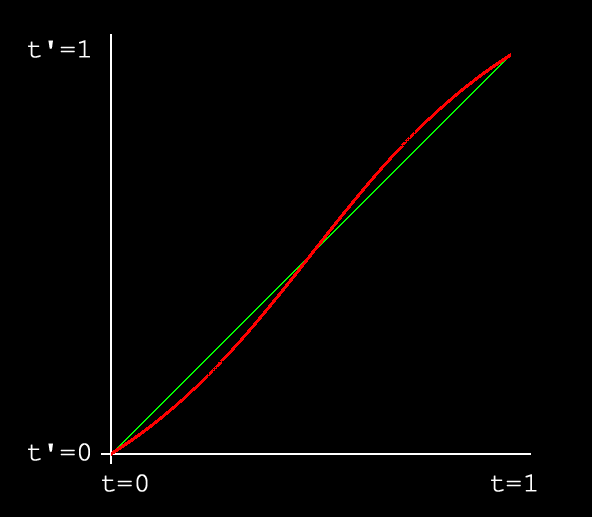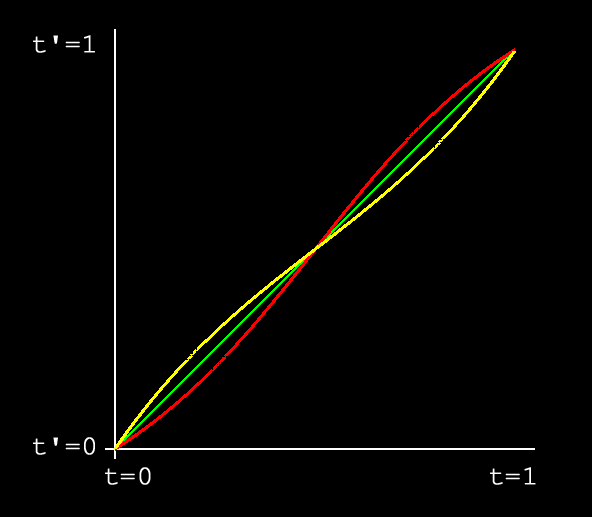Figure 2: Worst case of lerp speed variation. Green: ideal result produced by slerp; Red: distorted result produced by lerp. The error between these two functions should be measured vertically, so they are more different than they may appear at first. Figure 3: The compensating cubic spline, k = 0.45, shown in yellow atop the graph of figure 2.

Augmenting Linear Interpolation

The linear interpolation is monotonic from q1 to q2, so if you are doing an application where you're binary searching for a result that satisfies some constraint, using the linear interpolation works just fine. If your quaternions are very close together (under 30 degrees, say), as you have when playing back a series of time-sampled animation data, linear interpolation works fine. And if you have some number of different character poses (like an enemy pointing his gun in several different directions), and you want to mix them based on a blending parameter, linear interpolation probably works fine.

Linear interpolation won't work if you need precise speed control and wide interpolation angles. But maybe we can fix that.

Perhaps we can make a spline that cancels most of the speed distortion. Looking at Figure 2, can we concoct a function that, when multiplied against the curve, causes it to lie much closer to the ideal line? The way I chose to visualize this was with a cubic spline that tries to pull the distortion function onto the diagonal. Figure 3 shows a cubic spline with the equation y = 2kt3 - 3kt2 + (1 + k)t, where the tuning parameter k = 0.45, graphed against the plot of figure 2.  [Note: We are looking for some function g to help us correct f; strictly speaking, the most general approach is to structure the correction as g(f(t)), not g(t)f(t).  But I can get away with thinking of this correction as a multiplication problem because: (1) In this case f(t) = t, and (2) I'm using splines with no constant coefficients, so I can factor t out of the spline.  Essentially I used some foreknowledge about the nature of the solution to simplify the problem.]

Because both the distortion curve and our compensating spline have an average value of 't', and are approximately complementary, when we multiply them together we get a function that is approximately g(t) = t2. We want g(t) = t, so we'll divide the cubic spline by t. Fortunately, since the spline passes through the origin, it has no 'd' coefficient; so dividing by t just turns it into a quadratic curve: y = 2kt2 - 3kt + 1 + k.

So now, if we're linearly interpolating two splines that are 90 degrees apart, we find t' = 2kt2 - 3kt + 1 + k, and use t' as our interpolation parameter. We get something very close to constant-speed interpolation (I will quantify how close in a little bit). However, if we reduce the angle between the input quaternions, we get something that's less accurate than the original 't'.

That's because I concocted this spline specifically for the worst-case scenario, by defining its slope at t = 0 and t = 1. That's where the 'k' parameter comes in: it's a slope control mechanism. To get this spline to compensate for distortion across the full range of quaternion input angles, we want to adjust the tuning parameter as some easily-computable function of the angle between the two quaternions.

Well, taking the dot product of two quaternions gives us cos α, the cosine of the angle between them. I started playing around with simple functions of cos α until I found something reasonable. Basically, we want a function that is 1 when cos α = 0, and that is near 0 when cos α = 1. After some experimentation I landed on k = 0.45 (1 - s cos α)2, where s = 0.9 for now. To cursory visual inspection, this gives pretty good results across the full range of α from 0 to 90 degrees.

These numbers are in the right neighborhood, but because I just made them up, they're not going to be as close as we can get. So I wrote some code to do hillclimbing least-squares minimization. The initial distortion function has an RMS error of about 1.6 x 10-2 when averaged over all interpolation sizes (the worst case, graphed in figure 2, has an RMS error of 3.234 x 10-2). The minimzier gave me the following values: k = 0.5069269, s = 0.7878088 yields an overall error of 2.07 x 10-3, which is about 8 times lower than we'd started with. See Listing 1.

 Listing 1: A function that splines 't' to compensate for the distortion induced by lerping. float correction(float t, double alpha, double k, double attenuation) {     double factor = 1 - attenuation * cos(alpha);     factor *= factor;     k *= factor;     float b = 2 * k;     float c = -3 * k;     float d = 1 + k;     double tprime_divided_by_t = t * (b*t + c) + d;     return tprime_divided_by_t; }

But while I had been aligning things by eye, I noticed that if I gave k a high value, I got results that were close to exact from t = 0 to t = 0.5, but diverged after t = 0.5. So I wrote an interpolator that only needs to evaluate t from 0 to 0.5. If you pass in a t higher than 0.5, it just swaps the endpoints of interpolation. Running the optimizer on this, I got k = 0.5855064, s = 0.8228677, overall error 5.85 x 10-4 -- a reduction of more than 27 from the original. We incur another small cost to gain this accuracy, an extra 'if' statement.

You can probably do better than these numbers; my methods were ad-hoc, and there are many possibilities I haven't explored. I should also give a few warnings, for example, the 'if' statement mentioned above introduces a slight discontinuity at t = 0.5; you can fix this discontinuity by shifting the midpoint away from .5 but this wasn't important for my needs.

So we can interpolate pretty quickly now, but we end up with non-unit quaternions. Probably we want unit quaternions, so how do we normalize without doing a really slow inverse sqrt operation?

Normalization

To normalize any vector, quaternions included, we want to divide the vector by its length. The squared length of some vector v is cheap to compute -- it's v·v -- so we need to obtain 1/sqrt(v·v) and multiply the vector by that. Division and square-rooting are pretty expensive though.

We can compute a fast 1/sqrt(x) by using a tangent-line approximation to the function. This is like a really simple 1-step Newton-Raphson iteration, and by tuning it for our specific case, we can achieve high accuracy for cheap. (A Newton-Raphson iteration is how specialized instruction sets like 3DNow and SSE compute fast 1/sqrt).

The basic idea is that we graph the function 1/sqrt(x), locate some neighborhood that we're interested in, and pretend that the function is linear there. A linear function is cheap to evaluate.

So: we want to approximate f(x) = 1/sqrt(x). We are interested in vectors whose lengths are somewhere near 1, meaning f(x) = 1, which means x = 1. So we are going to focus on the neighborhood x = 1, as you see in Figure 4. To get the line, we just take the derivative of f, f'(x) = -1/2 x-3/2, and evaluate it at 1: f'(1) = -1/2.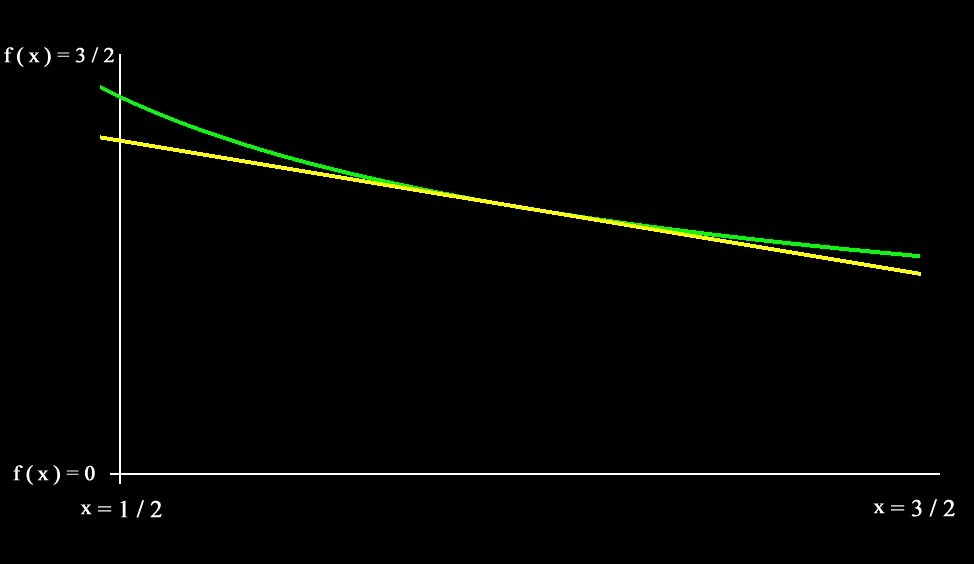Figure 4: In green, the function f(x) = 1/sqrt(x); in yellow, its tangent line at x = 1.

An equation that says "locally, a function is approximately its value at some point plus its first derivative extrapolated over distance" is: f(x + Δx) f(x) + Δx f'(x). We evaluate this at x=1 to get f(1 + Δx) ~~ f(1) + Δx f'(1) = 1 - 1/2 Δx.

Now for the last trick: we want to represent the squared length of our input vector, which we'll call s, as a value in the neighborhood of 1, so we can plug it into our new linear function. We say s = 1 + Δx, and thus Δx = s - 1.

That is all we need. When we plug Δx = s - 1 into our approximation, we get f(1 + s - 1) 1 - 1/2(s - 1). Simplified, this says: f(s) 1/2(3 - s). For as wide of a neighborhood as 1/sqrt is well-approximated by a tangent line, this extremely fast computation will give us the factor to normalize a vector. Figure 5 graphs the vector lengths we get when we use this computation to normalize. As long as we start with a vector whose length is near 1, we get results that are fairly accurate.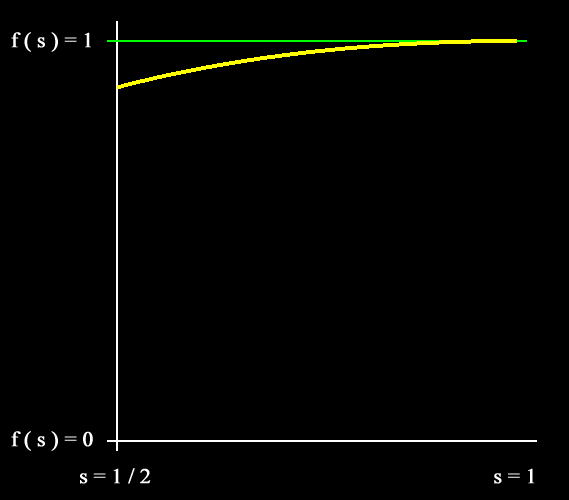Figure 5: The length of an approximately normalized vector (yellow), versus the squared length of the input, when using the naive tangent line approximation. The green line indicates the ideal result.

For some applications, accuracy in a narrow range is all we need. If you are reconstructing quaternions from splines, as one might do in a skeletal animation system that stores animation data in a small memory footprint, you can ensure a maximum length deviation during the spline-fitting process (inserting extra keyframes to alleviate any problems). Then at runtime you just evaluate the splines and pump the coefficients into this one-step normalizer, and you can be assured that the results are good.

On the other hand, this isn't good enough to use blindly on the results of quaternion linear interpolation. We can see that, during our worst-case interpolation from (1, 0) to (0, 1), the closest we get to the origin is (1/2, 1/2), which gives us a squared vector length s = 1/2. So for good results after lerping, we need a fast normalizer that produces good results all the way through the interval from s = 1/2 to s = 1.

Re-Tuning the Tangent Line Approximation

When we linearly interpolate quaternions, we get a chord that cuts through the unit sphere; that is, the resulting length is always less than 1. So we don't need our linear approximation to be accurate above 1. We can, in effect, slide the graph of figure 5 to the left a little bit, making our approximation more effective for shorter vectors.

Also, if we are going to permit some small amount of error ε in our result, it probably makes sense to allow results in the range 1 ± ε, instead of just 1 - ε as in figure 4. So we can scale the approximation by some small factor. This roughly doubles the zone of good results.

But this still doesn't cover the full range from 1/2 to 1, so what do we do if we need to handle all that? A simple idea would be to just check the value of 's', and if it is too low, just compute the answer the slow way. For most applications, wide-angle interpolations will be extremely rare so the speed hit will be small. But if you need to be faster than that, there are some hackish things we can do.

I wrote some code that repeatedly applies the fast normalization, tuned by some optimization parameters, in order to achieve the least error across the interval we are interested in (Listing 2). Running the numerical optimizer on this yields x_offset = .959066, scale = 1.000311, and a root-mean-square error of 2.15 x 10-4... which seems pretty darn good. This loop only iterates at most 3 times over the interval we care about, so you can re-phrase the loop as a small series of nested 'if' statements, which are mostly never descended into (Listing 3).

 Listing 2: A loop that repeatedly applies the fast normalizer until an acceptable value is reached.  (This is an expanded version to facilitate experimentation; a production version would have a lot of constants factored out, like 1/sqrt(neighborhood).) inline double isqrt_approx_in_neighborhood(double s, double neighborhood, double scale) {     return scale / sqrt(neighborhood) + scale * (s - neighborhood) * (-0.5 * 1.0 / (neighborhood * sqrt(neighborhood))); } double fast_normalize(double s, double neighborhood, double scale) {     double factor = 1;     const double LIMIT = 0.9995;     double limit2 = LIMIT * LIMIT;     int ntries = 0;     while (factor * factor * s < limit2) {         double new_factor = fast_normalize(factor * factor * s,                                            neighborhood, scale);         factor *= new_factor;         // 'ntries' is just a mechanism to keep us from infinite-looping         // while we are experimenting.         ntries++;         if (ntries > 8) break;     }     return factor; } Listing 3: A possible production version of Listing 2. inline float isqrt_approx_in_neighborhood(float s) {     const float NEIGHBORHOOD = 0.959066;     const float SCALE = 1.000311;     const float ADDITIVE_CONSTANT = SCALE / sqrt(NEIGHBORHOOD);     const float FACTOR = SCALE * (-0.5 / (NEIGHBORHOOD * sqrt(NEIGHBORHOOD)));     return ADDITIVE_CONSTANT + FACTOR * (s - NEIGHBORHOOD); } inline void fast_normalize(float vector) {     float s = vector*vector + vector*vector + vector*vector;     float k = isqrt_approx_in_neighborhood(s);     if (s < 0.83042395) {         k *= isqrt_approx_in_neighborhood(s);         if (s < 0.30174562) {             k *= isqrt_approx_in_neighborhood(s);         }     }     vector *= k;     vector *= k;     vector *= k; }

Sample Code

This month's sample code implements fast linear interpolation and renormalization, as well as the numerical optimization code that computes the best parameters. See you next time.

References

"Animating Rotation with Quaternion Curves", Ken Shoemake, Computer Graphics V.19 N.3, 1985.
"Quaternion Algebra and Calculus", David Eberly. http://www.magic-software.com/Documentation/quat.pdf